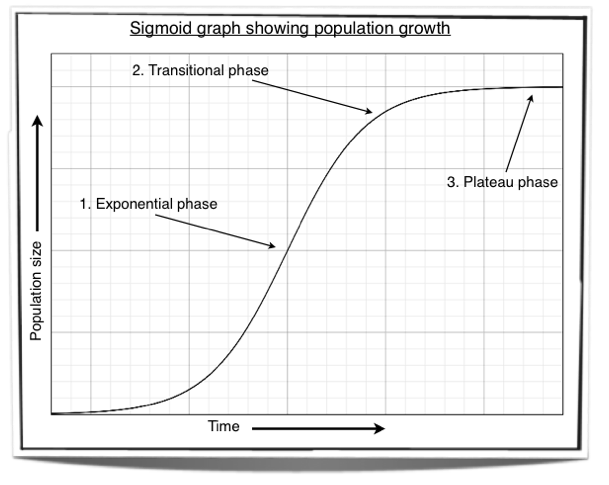# Populations

## 5.3.1 Outline how population size is affected by natality, immigration, mortality and emigration.

Natality: increases population size as offspring are added to the population.

Immigration: increases population size as individuals have moved into the area from somewhere else and so this adds to the population.

Mortality: decreases the population as some individuals get eaten, die of old age or get sick.

Emigration: decreases the population as individuals have moved out of the area to go live somewhere else.

## 5.3.2 Draw and label a graph showing a sigmoid (S-shaped) population growth curve.Figure 5.3.1 - A sigmoid population growth cruve

## 5.3.3 Explain the reasons for the exponential growth phase, the plateau phase and the transitional phase between these two phases.

The sigmoid graph showing the population growth of a species has three phases which are; the exponential phase, the transitional phase and the plateau phase. At the start of the sigmoid curve we can see the exponential phase. This is where there is a rapid increase in population growth as natality rate exceeds mortality rate. The reason for this is because there are abundant resources available such as food for all members of the population and diseases as well as predators are rare. As time passes, the population reaches the transitional phase. This is where the natality rate starts to fall and/or the mortality rate starts to rise. It is the result of a decrease in the abundance of resources, and an increase in the number of predators and diseases. However, even though population growth has decreased compared to the exponential phase, it is still increasing as natality rate still exceeds mortality rate. Finally, the population reaches the plateau phase. Here, the population size is constant so no more growth is occurring. This is the result of natality rate being equal to mortality rate and is caused by resources becoming scarce as well as an increase in predators, diseases and parasites. These are the limiting factors to the population growth. If natality rate starts to drop then mortality rate will drop too as more resources become available. As natality rate starts to increase again so does mortality rate as resources become scarce. This keeps the population number relatively stable. If a population is limited by a shortage of resources then we say that it has reached the carrying capacity of the environment.

Summary:

Exponential phase:

1. Rapid increase in population growth.
2. Natality rate exceeds mortality rate.
3. Abundant resources available. (food, water, shelter)
4. Diseases and predators are rare.

1. Natality rate starts to fall and/or mortality rate starts to rise.
2. There is a decrease in the number of resources.
3. An increase in the number of predators and diseases.
4. Population still increasing but at a slower rate.

Plateau phase:

1. No more population growth, population size is constant.
2. Natality rate is equal to mortality rate.
3. The population has reached the carrying capacity of the environment.
4. The limited resources and the common predators and diseases keep the population numbers constant.

## 5.3.4 List three factors that set limits to population increase.

1. Shortage of resources (e.g. food)
2. Increase in predators
3. Increase in diseases and parasites